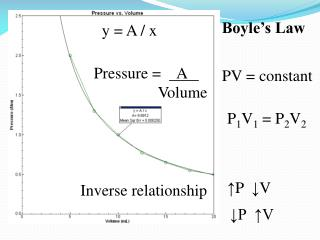DownloadDownload PresentationP 1 V 1 = P 2 V 2

# P 1 V 1 = P 2 V 2

Download Presentation## P 1 V 1 = P 2 V 2

- - - - - - - - - - - - - - - - - - - - - - - - - - - E N D - - - - - - - - - - - - - - - - - - - - - - - - - - -
##### Presentation Transcript

1. Boyle’s Law y = A / x Pressure = A Volume PV = constant P1V1 = P2V2 ↑P ↓V Inverse relationship ↓P ↑V

2. Practice Problem A gas occupies a volume of 3.86 L at 0.750 atm. At what pressure will the volume be 4.86 L? V1 P1 ? P2 V2 P1V1 = P2V2 (0.750 atm)(3.86 L) = P2(4.86 L) (0.750 atm)(3.86 L) = P2 (4.86 L) = 0.596 atm

3. Charles’Law V= constant T y = mx + b V1=V2 T1 T2 K = 0C + 273 Direct relationship ↑T ↑V -273

4. Practice Problem A 4.50 L container of nitrogen gas at 28.0 0C is heated to 56.0 0C. Assuming the volume of the container can vary, what is the new volume of the gas? T1 V1 T2 ? V2 V1=V2 T1 T2 4.50 L=V2 301 K 329 K K = 0C + 273 V2 = 4.92 L

5. Gay-Lussac’s Law y = mx + b P= constant T P1= P2 T1 T2 K = 0C + 273 Direct relationship -273 0C ↑T ↑P

6. Practice Problem A gas cylinder contains 40.0 L of gas at 45.0 0C and has a pressure of 650. torr. What will the pressure be if the temperature is changed to 100. 0C? T1 ? P2 P1 T2 P1= P2 T1 T2 650. torr = P2 318 K 373 K K = 0C + 273 P2 = 762 torr

7. P, V PV = constant Combined Gas Law P, T P = constant T PV = constant T V, T V = constant T P1V1 = P2V2 T1 T2 K = 0C + 273 STP = Standard Temperature & Pressure 00C, 273 K 1 atm

8. Practice Problem 15.00 L of gas at 45.0 0C and 800. torr is heated to 400.0C, and the pressure changed to 300. torr. What is the new volume? V1 T1 P1 P2 T2 ? V2 P1V1 = P2V2 T1 T2 K = 0C + 273 (800. torr)(15.00 L) = (300. torr) V2 318 K 673 K V2 = 84.7 L

9. Practice Problem To what temperature must 5.00 L of oxygen at 50. 0C and 600. torr be heated in order to have a volume of 10.0 L and a pressure of 800. torr? ? T2 V1 T1 P1 V2 P2 P1V1 = P2V2 T1 T2 K = 0C + 273 (600. torr)(5.00 L) = (800. torr)(10.0 L) 323 K T2 T2 = 861 K = 588 0C

10. Do problems: 1 & 2 pg 443 4-6 pg 446 8 & 9 pg 448 11& 12 pg 450